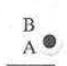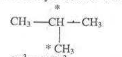# NDA 2012 CHEMISTRY Past Questions

Scroll Down and click on link or Go to Link for destination

1. Sieving is a technique used to separate mixtures containing solid particles of___.
A. small sizes
B. large sizes
C. different sizes
D. the same sizes

2. Which of the following compounds is composed of Al, Si, 0 and H?
A. Epsom salt
B. Limestone
C. Clay
D. Urea

3. Calculate the molarity of a solution containing 7.0g of potassium chloride in 1dm(K = 39. Cl = 35.5)
A. 0.011M KCl
B. 0.045M KCl
C. 0.094M KCI
D. 0.055M KCI

4. In the titration of oxalic acid against sodium hydroxide, the best indicator will be ___.
A. methyl orange
B. phenolthalein
C. phenolphthalein
D. litmus

5. Hydrogen bomb is based on the principle of ___.
A. nuclear fission
C. nuclear fusion

6. The IUPAC name of coordination compound
K3[Fe(CN)6] is
A. potassium hexalyanoferrate II
B. potassium hexalyanoferate III
C. potassium hexalyanoiron II
D. tripotassium hexalyaniron II

7. The nucleus of an atom consists of ___.
A. electrons and neutrons
B. electrons and protons
C. proton and neutrons
D. All of the above

8. The law which states that the amount a gas dissolved in a liquid is proportional to its partial pressure is ___.
A. Dalton's Law
B. Gay Lussac's Law
C. Henry's Law
D. Raoultis Law

9. Below is a paper chromatogram of a mixture of dyes:Point of application

Which dye is least absorbed by the paper?
A. A
B. C
C. D
D. B

10. A hydrocarbon contains 20 by mass of hydrogen. What is its empirical formula?
A. CH2
B. CH3
C. C2H6
D. CH

11. Which of the following is NOT true for sigma (σ) bond?
A. It is stronger than pi (a) bond
B. It is weaker than pi (n) bond
C. It is formed as a result of head-to-head overlap of two atomic orbitals at 180˚
D. It results in the formation of single bond

12. What is the hybridization of the carbon atoms marked with asterisk?A. SP3 and SP2
B. SP2 and SP3
C. SP3 and SP3
D. SP and SP2

13. Which of the following is an aromatic compound?
A. Cyclohexane
B. Cyclobutane
C. Benzene
D. Methanol

14. One of the main points of Dalton's Atomic Theory can be stated as follows:
A. Atoms of the same elements can combine to form bridged mixture of compounds
B. Atoms of different elements can combine to form compound of polymers
C. Atoms of different elements can be combined to form complex ratios to each other
D. Atoms of different elements combine to form a compound, the number of atoms combined hearing a simple while number ratio to each other

15. Which of the following is true?
A. Standard enthalpy change of combustion is the enthalpy change when two moles a substance is completely ashed in nitrogen
B. Standard enthalpy change of combustion is the enthalpy change when one mole of the substance. is burnt completely in oxygen under standard conditions
C.  Standard enthalpy change of combustion is the enthalpy change when one mole of oxygen and methane are liquefied in Nitrogen under standard conditions
D. Standard enthalpy change of combustion is enthalpy change when one mole of oxygen reacts endothermically with other substances under standard conditions

16. An increase in the concentration of reactants will increase the reaction rate. This can be explained by:
A. the plumber of effective collisions between the reactant particles
B. the number of effective nuclear screening effects between the reactant particles
C. the number of effective mean free path of the compound in the system
D. the number of crowded substances in the gaseous form

17. Consider the reaction
N204(g)  2NO2(g)
colourless  ↔ dark brown

Which one of the following correctly describes what you would see if the pressure was decreased?
A. The gas mixture would become lighter
B. The gases would condense to liquids
C. The gas mixture would become dark brown
D. The gases would separate into two layers

18. The electronic configuration of the atom is ____.
A. 2, 8, 3
B. 1s2 2s2 2p6 3s2 3p1
C. 1s2 2s2 2p6 3s1
D. 2, 8, 2

19. Which one of the following is not true about chlorine water?
A. It can displace fluorine from a fluoride
B. It is used as a disinfectant
C. It can bleach litmus paper
D. It can displace bromine from a bromide

20. What is the oxidation number of chromium in Potassium Chromate (K2CrO4)
A. +4
B. +6
C. +8
D. -4

21. The solubility of solids increases with an increase in the temperature of solvent. Which of the following salts has its solubility unaffected with a rise in temperature?
A.   KNO3
B.   KI
C.   KCl
D.   NaCl

22. In the contact process for the manufacture of sulphuric acid, sulphur trioxide gas is not passed directly in water because:
A. It is not much soluble in water
B. It does not form sulphuric acid with water
C. Colloidal sulphur is deposited
D. A mist of fine chops of dilute sulphuric acid fill the pipes if direct adsorption in water is tried

23. Cu(s) + HNO3(l)
Cu(NO3)2(l) +H20(l) + 2NO2(g) in the above reaction, nitric acid is acting as:
A. an oxidizing agent
B. a reducing agent
C. a nitrating agent
D. a hydrating agent

24. Which of the following salt is used in the preparation of caustic soda, washing soda, hydrochloric acid, bleaching powder etc?
A. NaNO3
B. Na2O
C. NaSO4
D. NaCl

25. Ammonia is used as a commercial refrigerant because:
A. it is a non-polar compound
B. the gas is very cheap
C. it is very soluble in water
D. it is easily liquefied and when allowed to evaporate, absorbs large, quantities of heat producing low temperature

26.If a salt is dissolved in water and the resulting solutions feels cold to the hand. This implies that it is:
A. Thermostatic
B. Thermophilic
C. Exothermic
D. Endothermic

27. Which of the following statements is corrects about Osmotic Pressure?
A. It is the pressure required to prevent a solute flowing across a semi-permeable membrane from high to low region of concentration
B. It is the pressure required to allow a solvent flowing across a semi-permeable membrane from high to low region of concentration
C. It is the pressure required to prevent a solvent flowing across a semi-permeable membrane from a region of high to low concentration
D. It is the pressure required to prevent a solvent flowing across a semi-permeable membrane from a region or low to high to concentration

28. The heat change associated with a given chemical change is always constant and is independent of time and of any intermediate changes involved. This is
A. Dalton's Law of Heat Summation
B. Hess's Law of Heat Summation
C. Dulon & Petil Law of Heat Summation
D. Born-Haber Law of Heat Summation

29. Electrovalency is best favoured by:
A. a large metallic atom and a small non-metallic atom
B. a small stable atom
C. a large anion which loses electrons
D. resonance in the bonding pairs

30. Which of the following better defines a black body?
A. A perfect absorber of energy and retains any radiant energy
B. A perfect absorber of transmitted energy
C. A perfect absorber of monochromatic light
D. A perfect absorber of quantised energy

A. melting point is increased
B. workability is reduced
C. the alloy is used in joining metals
D. the alloy is a catalyst in iron are extraction

32. Which of these ions gives a dark green precipitate with sodium hydroxide solution but a dark blue precipitate with potassium hexacyanoferrate (ii) Solution?
A. Fe3+
B. Fe2+
C. Pb2+
D. Sn2+

33. What is the effect of addition of a catalyst on a reversible reaction in a dynamic equilibrium?
A. shifts the equilibrium to the right
B. shifts the equilibrium to the left
C. does not affect the equilibrium position
D. increase the equilibrium constant

34. Which of these statements is true?
A. A base is always soluble in water
B. Sodium hydroxide is both a base and an alkali
C. Hydrogen is released whenever an acid and metal are mixed
D. A gardener neutralizes an alkaline soil by the addition of lime

35. When the trixonitrate (v) salt of an alkali metal M is heated. the formula of the solid product formed is ___.
A. M2O3
B. MnO
C. MO2
D. MnO2

36. Chromatograph is used to separate components of mixtures which differ in their rates of ___.
A. diffusion
B. migration
C. reaction
D. sedimentation

37. The number of hydrogen ions in 4.8g of tetraoxosulphate (V) acid is
A. 3.01 x 1022
B. 6.02 x 1023
C. 3.01 x 1023
D. 6.02 x 1023
[S= 32, O= 16, H=1] Na = 6.02 x 1023]

38. Which of the following tests will completely identify any one of sulphur (IV) oxide, hydrogen, carbon (IV) oxide and nitrogen (II) oxide?
A. pass each gas into water and test with blue litmus paper
B. pass each gas into lime water
C. expose each gas to atmosphere air
D. pass each gas into concentrated tetraoxosulphate (VI) acid

39. In the Haber process for the manufacture of ammonia, the catalyst commonly used is finely divided ___.
B. platinum
C. iron
D. copper

40. A metallic oxide which reacts with both HCl and NaOH to give salt and water only can be classified as ___.
A. an acidic oxide
B. a basic oxide
C. a neutral oxide
D. an amphoteric oxide

1. C
2. C
3. C
4. C
5. C
6. B
7. C
8. C
9. A
10. B
11. B
12. C
13. C
14. D
15. B
16. A
17. D
18. D
19. A
20. B
21. D
22. D
23. A
24. D
25. D
26. D
27. C
28. B
29. A
30. A
31. C
32. B
33. C
34. B
35. D

Share This Post: If you believe this post will be useful to others, please share it using the buttons below!